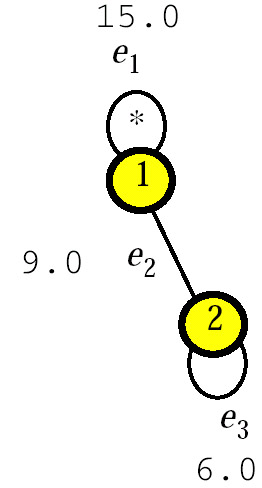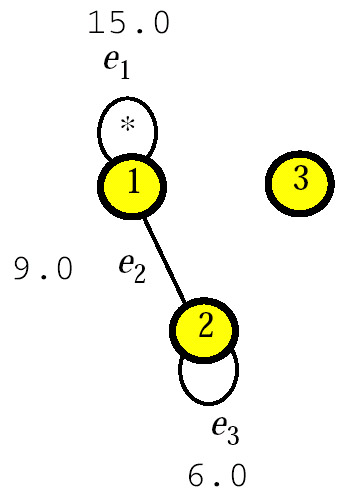# AN AUDITING PROCEDURE

In this section we sketch an auditing procedure, which given

• a sensitivity criterion, specified by a "sensitivity test";

• a safety criterion, specified by a "safety test," which given the data map of a query set Q, correctly decides whether Q is or is not safe;

• a safe set of queries {Q1,, Qn}, specified by its data map (H, w), where H = (V, E) with V = {1,, n} and E = {e1,, em}; and

• a new query Qn+1 specified by a subset B of the domain of the category variable and with value Qn+1

computes the value of a Boolean variable safe, which will come out to be True if and only if Q n+1 can be safely answered. The procedure is as follows.

• Step 1. Set safe := False.

• Step 2. Apply the sensitivity test to Qn+1. If Qn+1 turns out to be sensitive, then Exit.

• Step 3. If Qn+1 belongs to the closure of {Q1,, Qn}, then set safe := True and Exit.

• Step 4. Construct the data map (H, w) of {Q1,, Qn, Qn+1}.

• Step 5. If no edge of H is marked, then set safe := True and Exit.

• Step 6. Apply the safety test to {Q1,, Qn, Qn+1}. If {Q1,, Qn, Qn+1} turns out to be safe, set safe := True.

The implementation of Steps 1, 2, 5, and 6 are straightforward. The problem of computing the closure of a query set was discussed earlier. We now detail Step 4, that is, how the data map (H, w) of {Q1,, Qn, Qn+1} can be constructed from the data map (H, w) of {Q1,, Qn}. Let V and E be the vertex set and the edge set of H, respectively. Recall that each edge e of H is labeled by a class, denoted by label[e], of the characteristic partition of the active domain of the category variable with respect to {Q1,, Qn}. For each edge e of H, by label[e] we denote the class of the characteristic partition of the active domain of the category variable with respect to {Q1,, Qn, Qn+1}.

` (4.1) Set V' := V ⋓ {n+1}, E' := E, and m' := m. (4.2) For k = 1,..., m, if B ∩ label[ek] = Ø then set w'(ek)       := w(ek) and label'[ek] := label[ek]; otherwise, do:       begin                        if label[ek] ⊆ B then do:       begin        ek := ek ∪ {n+1};       B := B - label[ek], qn+1 := qn+1 - w(ek)       end; otherwise, do:       begin       label'[ek] := label[ek] - B;       compute the value of Q(label'[ek]) and set w'(ek) to it;       apply the sensitivity test to Q(label'[ek]) and mark ek if Q(label'[ek]) turns       out to be sensitive;       m' := m'+1, em' := ek ∪ {n+1};       add em' to E';       label'[em'] := B ∩ label[ek]; B := B - label'[em'];       if B = Ø then set w'(em') := an+1 and Exit;       otherwise, do:             begin             compute the value of Q(label'[em']) and set w'(em') to it;             apply the sensitivity test to Q(label'[em']) and mark em' if             Q(label'[em']) turns out to be sensitive;             qn+1 := qn+1 - w'(em')             end       end end (4.3) Set m' := m'+1, em' := {n+1};       add em' to E';       label'[em'] := B;       w'(em') := qn+1;       apply the sensitivity test to Q(label'[em']) and mark em' if Q(label'[em'])       turns out to be sensitive. `

Example 11: Consider again the sum queries Q1, Q2, and Q3 of Example 2. Suppose we are given the data map of {Q1, Q2}, which is shown in Figure 35.Figure 35

Here, edge e1 = {1} is marked because the corresponding query is sensitive. The other two edges, e2 = {1, 2} and e3 = {2}, are not marked. The labels of these edges are label[e1] = {Direction}, label[e2] = {Administration} and label[e3] = {Services}.

We now apply the procedure above to construct the data map of {Q1, Q2, Q3} from the data map of {Q1, Q2}. After executing Step 4.1, the current value of (H, w) is as shown in Figure 36.Figure 36

During the execution of Step 4.2, edge e2 is not modified, but edge e1 will contain both vertex 1 and the vertex 3, and edge e3 will contain both vertex 2 and vertex 3. The data map of {Q1, Q2, Q3} is then as shown in Figure 32. Step 4.3 is not executed for B = Ø.

From a computational point of view, the worst case of Step 4 occurs if, when Step 4.2 is performed, B label[ek] Ø and neither label[ek] B nor B label[ek] for each k, 1 d k d m; then, the procedure has to evaluate 2m queries and apply the sensitivity test to each of them. To avoid such an overhead, we may introduce a query-overlap restriction (Malvestuto & Moscarini, 1999), according to which the query Qn+1 is left unanswered whenever during the execution of Step 4 an edge with more than two vertices is created. Accordingly, the data map of every set of answered queries is always a graph so that at Steps 3 and 6 we can also use the efficient algorithms for graphical query sets we reported in earlier sections.

Before closing this section, we wish point out a simple technique to reduce the size of a data map (H, w). It consists of deleting each vertex that is contained in exactly one edge of H. If H = (V, E) is the resulting hypergraph and w is the restriction of w to E, then it should be clear that, since no edge in EE can be marked in H (for, otherwise, a query in Q would be sensitive), the edge-weighted hypergraph (H, w) is equivalent to (H, w) from the point of view of safety, and one can test weak and strong safety using (H, w) instead of (H, w). We call the edge-weighted hypergraph (H, w) the reduced data map of Q.Multidimensional Databases: Problems and Solutions
ISBN: 1591400538
EAN: 2147483647
Year: 2003
Pages: 150